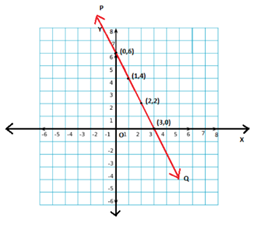# Linear Equations in Two Variables Class 9 Notes

## CBSE Class 9 Maths Linear Equations In Two Variables Notes:-

Get the complete notes on linear equations in two variables class 9 here. In this article, you are going to study the basics of linear equations involving one variable, two variables, and so on. Also, learn how to graph the linear equations, and how to find the solutions for linear equations in detail.

### Linear equation in one variable

When an equation has only one variable of degree one, then that equation is known as linear equation in one variable.

• Standard form: ax+b=0, where a and b ϵ R & a ≠ 0
• Examples of linear equation in one variable are :

– 3x-9 = 0
– 2t = 5

To know more about Linear equations in one variable, visit here.

### Linear equation in 2 variables

When an equation has two variables both of degree one, then that equation is known as linear equation in two variables.

Standard form: ax+by+c=0, where a, b, c ϵ R & a, b ≠ 0
Examples of linear equations in two variables are:
– 7x+y=8
– 6p-4q+12=0

To know more about Linear equations in 2 variables, visit here.

## Examples of Linear Equations

### The solution of linear equation in 2 variables

A linear equation in two variables has a pair of numbers that can satisfy the equation. This pair of numbers is called as the solution of the linear equation in two variables.

• The solution can be found by assuming the value of one of the variable and then proceed to find the other solution.
• There are infinitely many solutions for a single linear equation in two variables.

## Graph of a Linear Equation

### Graphical representation of a linear equation in 2 variables

• Any linear equation in the standard form ax+by+c=0 has a pair of solutions in the form (x,y), that can be represented in the coordinate plane.
• When an equation is represented graphically, it is a straight line that may or may not cut the coordinate axes.To know more about Graphical representation of a linear equation, visit here.

### Solutions of Linear equation in 2 variables on a graph

• A linear equation ax+by+c=0 is represented graphically as a straight line.
• Every point on the line is a solution for the linear equation.
• Every solution of the linear equation is a point on the line.

### Lines passing through the origin

• Certain linear equations exist such that their solution is (0, 0). Such equations when represented graphically pass through the origin.
• The coordinate axes namely x-axis and y-axis can be represented as y=0 and x=0, respectively.

### Lines parallel to coordinate axes

• Linear equations of the form y=a, when represented graphically are lines parallel to the x-axis and a is the y-coordinate of the points in that line.
• Linear equations of the form x=a, when represented graphically are lines parallel to the y-axis and a is the x-coordinate of the points in that line.

#### 1 Comment

1. thanks for the help